You can modify the display of a layer based on values of its attributes. Operations can be performed based on distinct or continuous values.

Here are some examples of classification based on the distinct values.

## Set a label and classification expression

The expression is an automatically generated field that contains rules to display objects on the map. While editing expression values make sure that you type exact value as written in the Data Grid. Note that features that don’t satisfy any condition will not be visible on the map.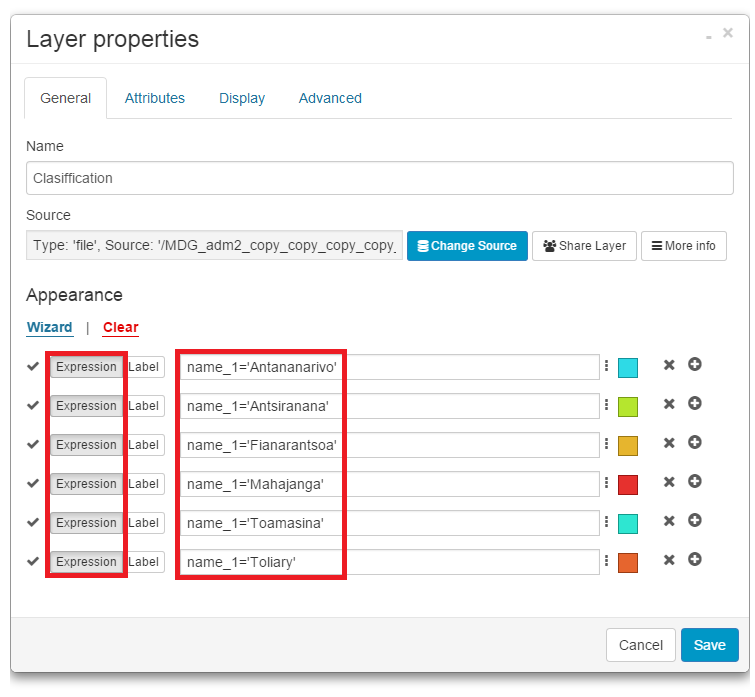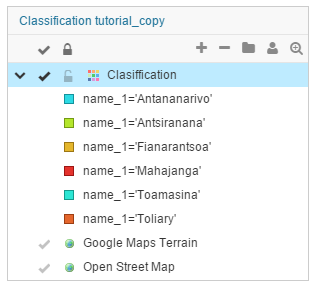If you want to rename a class, you can do it by clicking Label button in the Layer Properties. The label is class name visible in the Layer List. While renaming a class make sure that you are not in the “Expression” mode.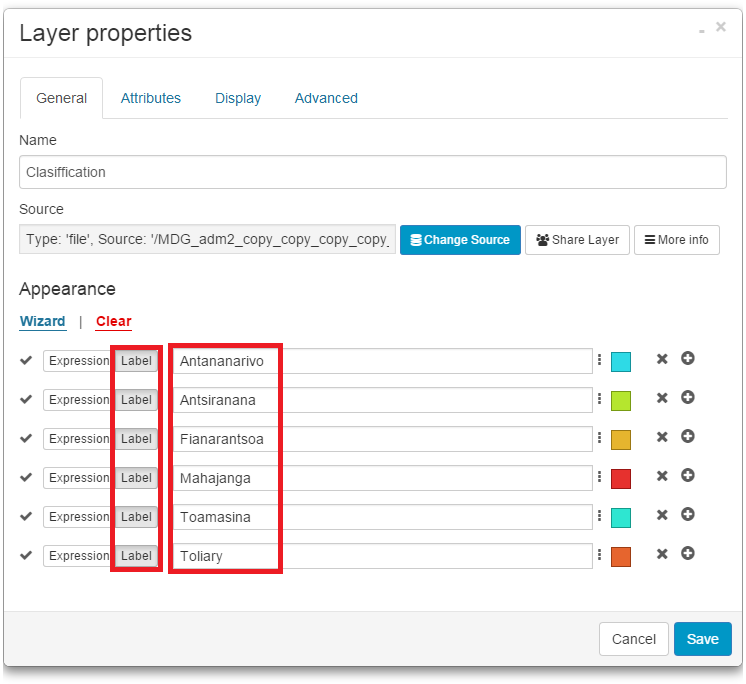### Example 1. Change a value of one class

1. Open a Layer Properties (Layer tab-Edit layer).
2. Click Wizard button.
3. Select the Distinct radio button.
4. Select the field whose values will define the layer display.
5. Click Calculate button.
6. Change a value of the expression.
7. Click Save.

Note: values are case sensitive.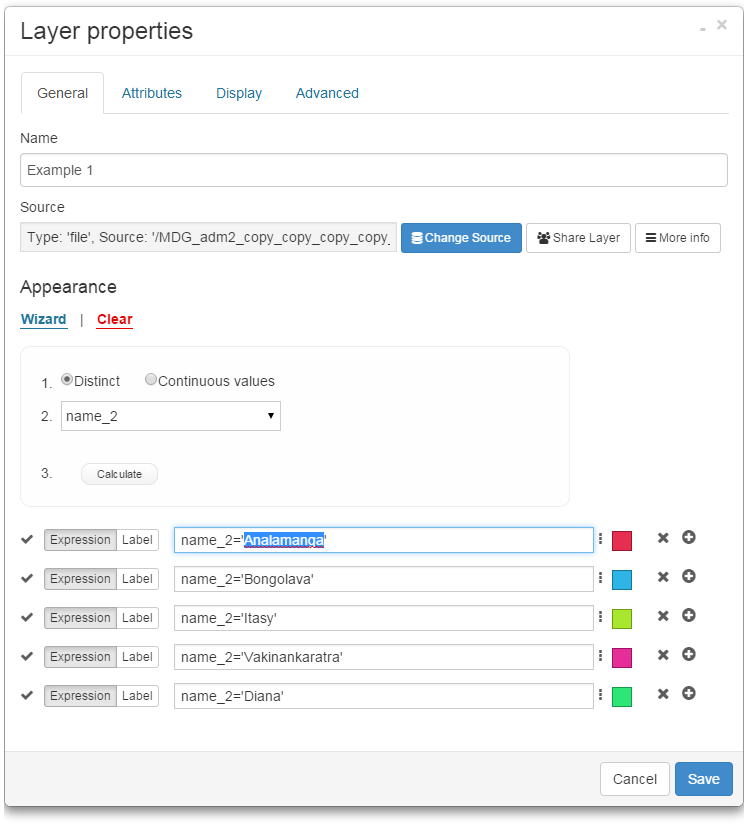### Example 2. Extract one class and put all other data into another class

It is possible to extract one class and put all other values into another class.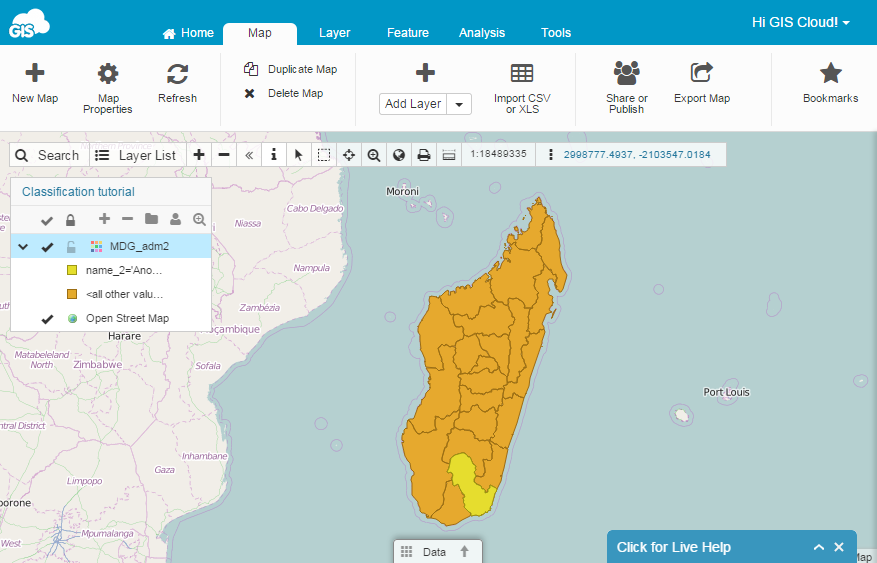1. Open a Layer Properties (Layer tab-Edit layer).
2. Click Wizard button.
3. Select the Distinct radio button.
4. Select the field whose values will define the layer display.
5. Click Calculate button.
6. In the first expression box, write the value that you want to extract (name_2=’Anosy’).
7. In the second expression box, enter 1.
8. Click Save button.

### Example 3. Display values of one class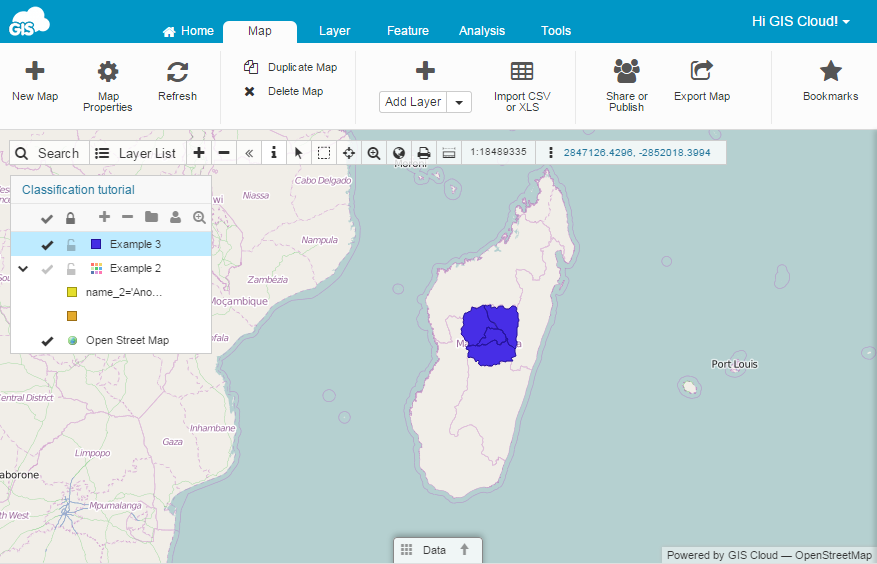1. Open a Layer Properties (Layer tab-Edit layer).
2. Click Wizard button.
3. Select the Distinct radio button.
4. Select the field whose values will define the layer display.
5. Click Calculate button.
6. In the expression box, enter the value that you want to extract (name_1=’Antananarivo’).
7. Delete all other classes.
8. Click Save.

### Example 4. Display more values in one class.

You can display more values in one class using the operator ‘OR’.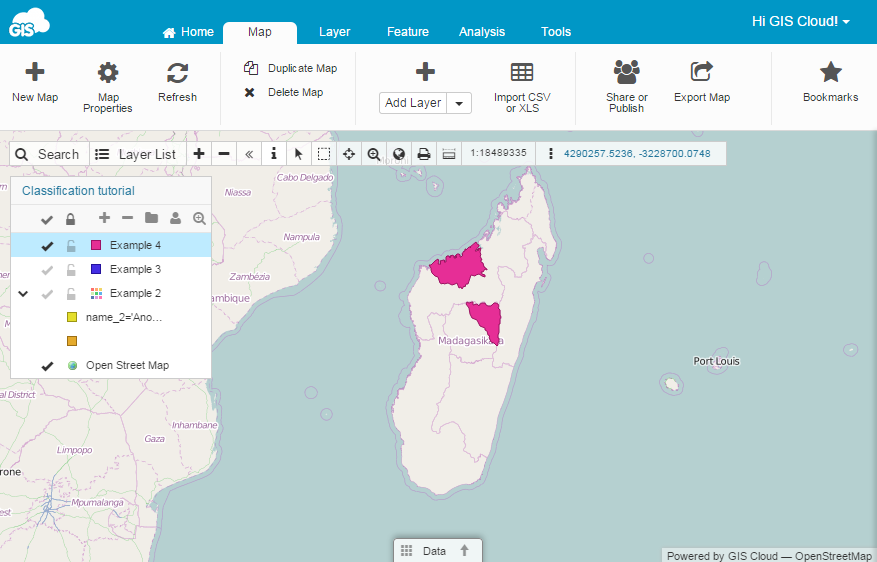1. Open a Layer Properties (Layer tab-Edit layer).
2. Click Wizard button.
3. Select the Distinct radio button.
4. Select the field whose values will define the layer display.
5. Click Calculate button.
6. In the expression box, write the first expression (name_2=’Analamanga’).
7. In the same expression box, write OR.
8. Write the second expression (name_2=’Boeny’).
9. Click Save.

### Example 5. More complex expression

Display features that contain value Analamanga and Ihorombe or if this feature doesn’t exist display features with value Bongolava.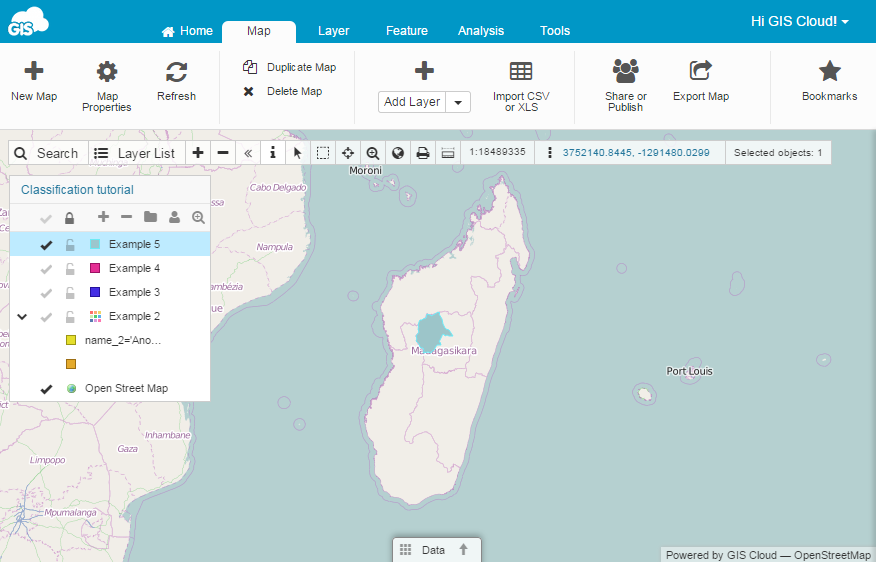1. Open a Layer Properties (Layer tab-Edit layer).
2. Click Wizard button.
3. Select the Distinct radio button.
4. Select the field whose values will define the layer display.
5. Click Calculate button.
6. In the expression box, write the first expression (name_2=’Analamanga’).
7. Write operator AND.
8. In the expression box, write the second expression (name_2=’Ihorombe’).
9. Write operator OR.
10. In the expression box, write the third expression (name_2=’Bongolava’).
11. Click Save button.

### Example 6. Display all classes except one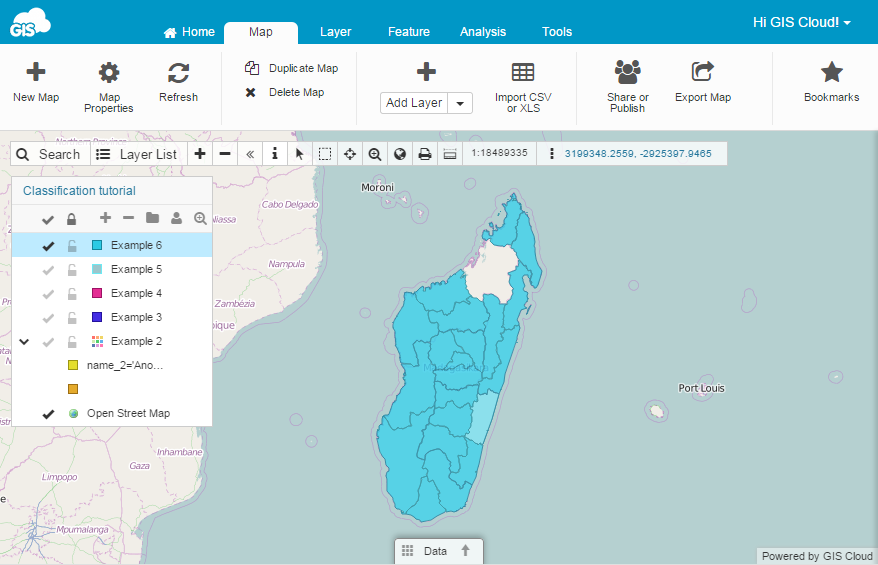1. Open a Layer Properties (Layer tab-Edit layer).
2. Click Wizard button.
3. Select the Distinct radio button.
4. Select the field whose values will define the layer display.
5. Click Calculate button.
6. In the expression box, write operator AND NOT.
7. In the expression box, write the expression that you don’t want to see (name_2=’Sofia’).
8. Click Save.